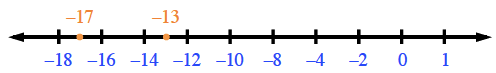### Home > CC1MN > Chapter 9 Unit 8 > Lesson CC3: 9.1.1 > Problem9-12

9-12.

Use $<, >,$or $=$ to compare the number pairs below.

1. $0.183 \text{ ____ } 0.18$

Write both numbers to the thousandths place, then compare.

1. $−13 \text{ ____} − 17$

It may help to place the numbers on a number line, like the one below.1. $0.125 \text{____}\frac { 1 } { 8 }$

Convert the fraction to a decimal, then compare.

1. $−6 \text{ ____} −4$

See part (b).

1. $72\%\text{ ____ }\frac { 35 } { 30 }$

Examine the fraction; is it greater than or less than 1?

1. $−0.25\text{ ____}−0.05$

See part (b).